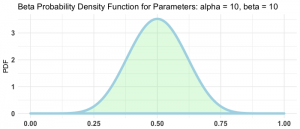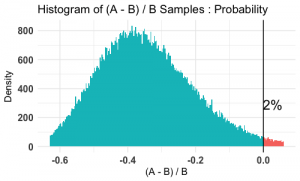Want to share your content on R-bloggers? click here if you have a blog, or here if you don't.

A/B Testing is a familiar task for many working in business analytics. Essentially, A/B Testing is a simple form of hypothesis testing with one control group and one treatment group. Classical frequentist methodology instructs the analyst to estimate the expected effect of the treatment, calculate the required sample size, and perform a test to determine if a large enough effect is observed. This method is somewhat lacking: it only leaves one with point estimates for the control and the treatment groups, and a verdict to reject (effect is observed) or to fail to reject (effect is not observed).

Let’s consider an alternative approach following Bayesian methods with the `bayesAB` package. Suppose that we have the current version and a proposed version of a web page, each containing a button of interest, and we wish to determine whether the proposed version leads to more clicks on the button of interest. Currently, approximately half of all visitors click the button of interest. Suppose the proposed version of the web page is actually much worse and only 30 percent will click it.

To test this, we randomly assign some visitors to the current and other visitors to the proposed version. Since a visitor either clicks the button of interest or not, we can treat this as a Bernoulli random variable with parameter theta. For the control and the treatment groups, we will assign the same prior distribution on theta, e.g., a beta distribution with mean 0.5. You can think of this as the analog of the null hypothesis.Now, let’s simulate 20 observations for each group and compare the posterior probabilities for the control and treatment groups. The package automatically computes the probability that the mean of the treatment is greater than the mean of the control.

```# First collection
control_1 <- rbinom(20, 1, 0.5)
treatment_1 <- rbinom(20, 1, 0.3)

Learn more about probability functions in the online course Statistics with R – Advanced Level. In this course you will learn how to

work with different binomial and logistic regression techniques,
know how to compare regression models and choose the right fit,
and much more.

# First Analysis
test1 <- bayesTest(treatment_1, control_1, distribution = "bernoulli", priors = c("alpha" = 10, "beta" = 10))
print(test1)
summary(test1)
plot(test1)```

The treatment posterior distribution is in red and the control posterior is in green. After 40 observations in total, the posteriors have started to separate, and the probability that the treatment is less than the control is approaching 95 percent.

Let’s simulate 20 more observations for each group and compare.

```# Second Collection
control_2 <- rbind(control_1, rbinom(20, 1, 0.5))
treatment_2 <- rbind(treatment_1, rbinom(20, 1, 0.3))

# Second Analysis
test2 <- bayesTest(treatment_2, control_2, distribution = "bernoulli", priors = c("alpha" = 10, "beta" = 10))
print(test2)
summary(test2)
plot(test2)```We can see that with the additional 40 observations, the distributions have separated more, and the probability that the treatment is less than the control is 98 percent.

In addition to point estimates and a verdict, we have full distributions for each of the parameters, which makes computing prediction intervals, for instance, easy. There’s much more to explore in this package than can be covered in this tutorial, so try getting your hands dirty with a few examples contained in the documentation.